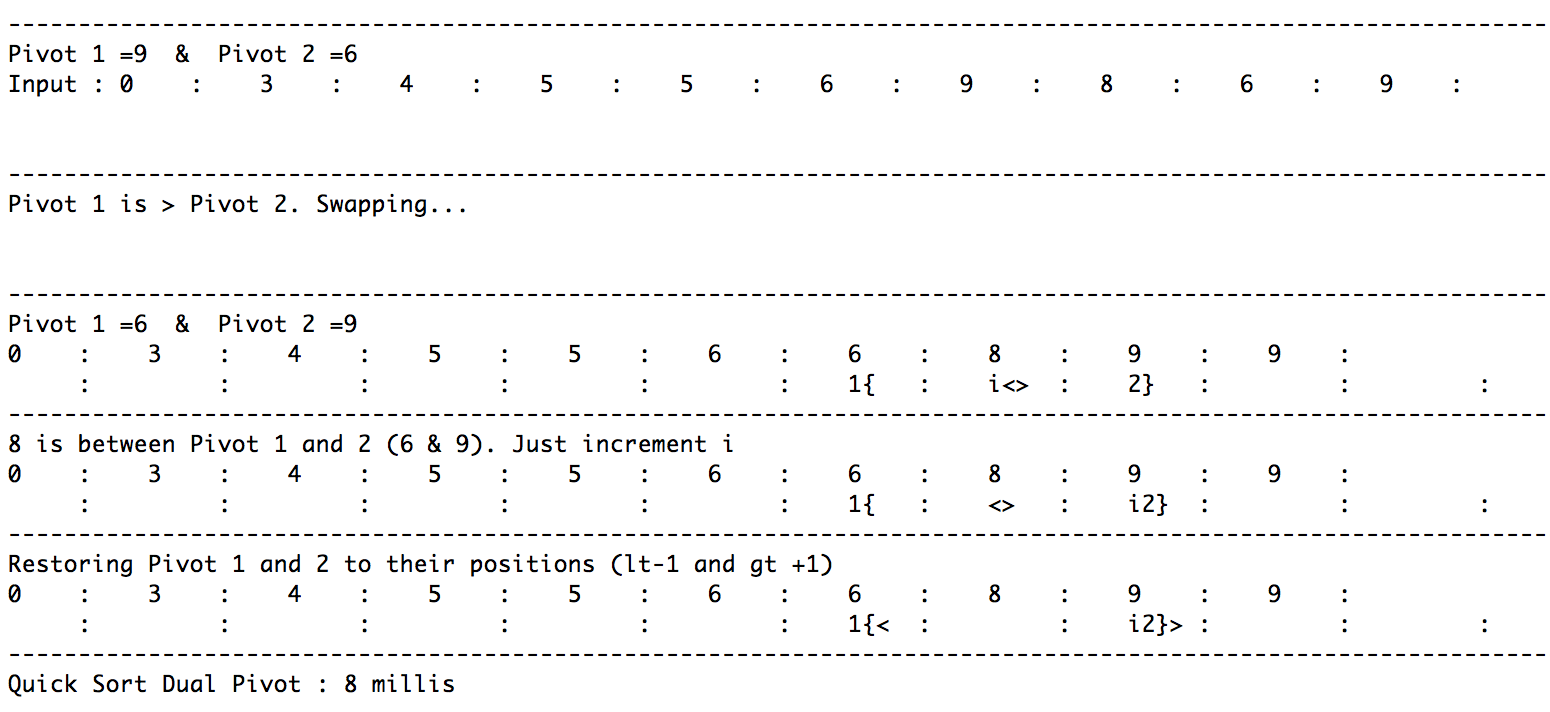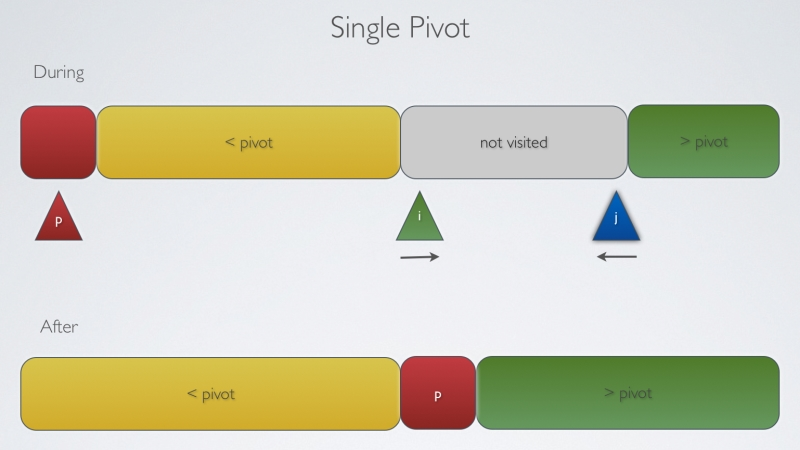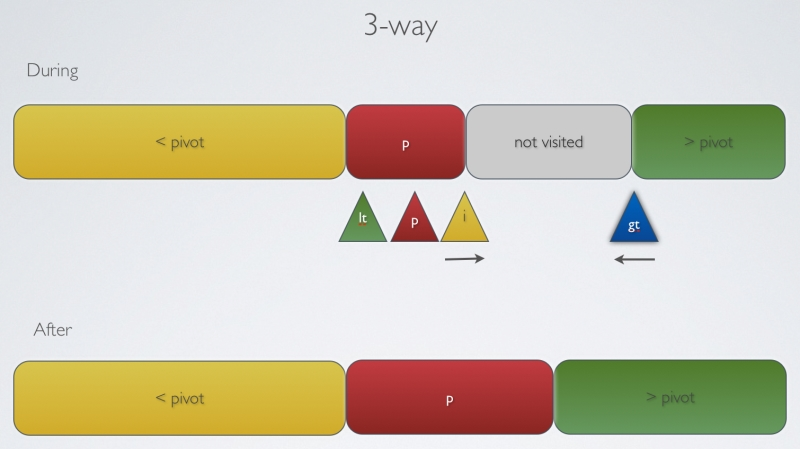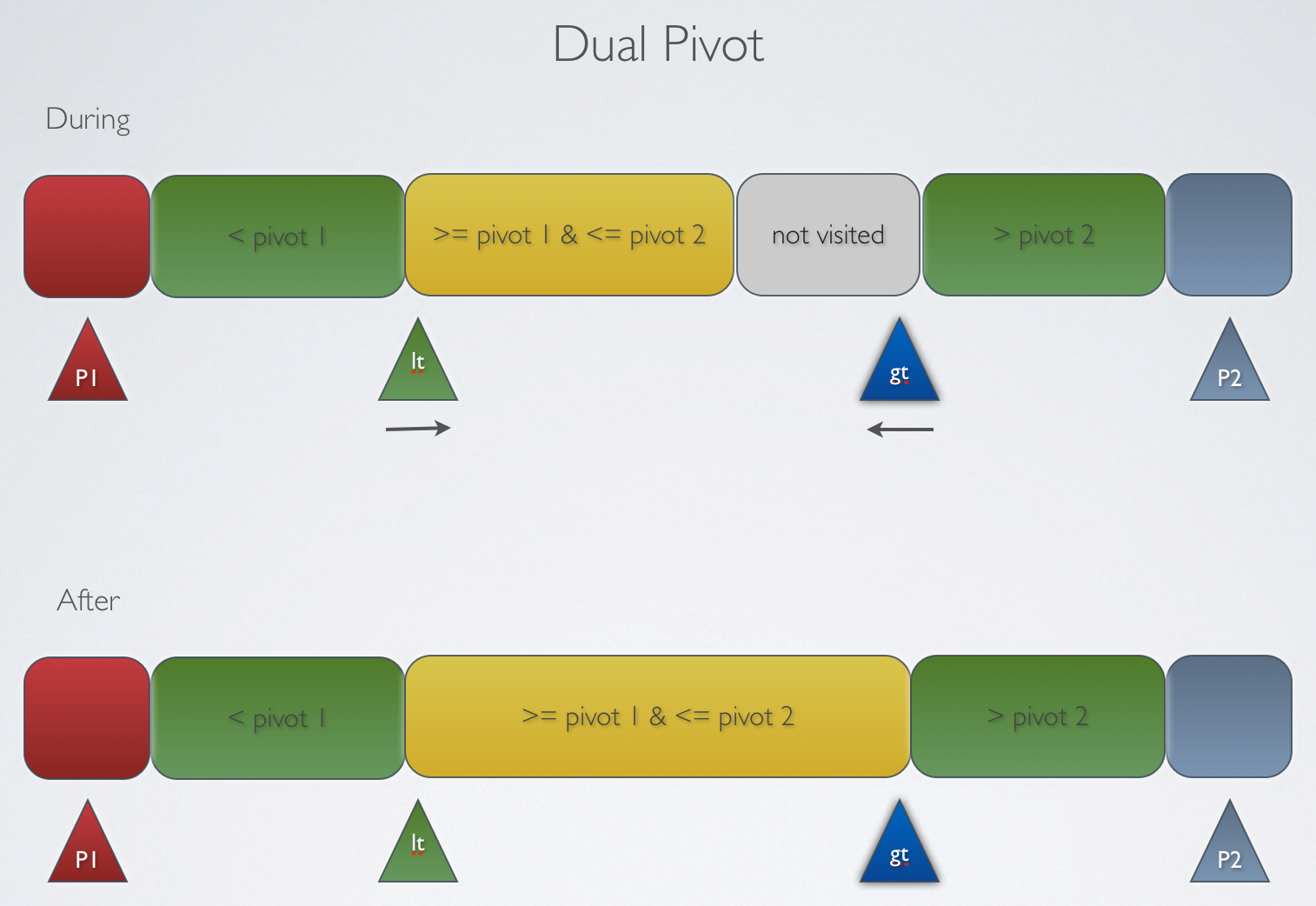It's no news that Quicksort is considered one of the most important algorithms of the century and that it is the defacto system sort for many languages, including the `Arrays.sort` in Java.

Well, nothing except that I figured just now (after 2 damn years of release of Java 7) that the Quicksort implementation of the `Arrays.sort` has been replaced with a variant called Dual-Pivot QuickSort. This thread is not only awesome for this reason but also how humble Jon Bentley and Joshua Bloch really are.

What did I do next?

Just like everybody else, I wanted to implement it and do some benchmarking - against some 10 million integers (random and duplicate).

Oddly enough, I found the following results :

Random Data :

• Quick Sort Basic : 1222 millis
• Quick Sort 3 Way : 1295 millis (seriously !!)
• Quick Sort Dual Pivot : 1066 millis

Duplicate Data :

• Quick Sort Basic : 378 millis
• Quick Sort 3 Way : 15 millis
• Quick Sort Dual Pivot : 6 millis

Stupid Question 1 :

I am afraid that I am missing something in the implementation of 3-way partition. Across several runs against random inputs (of 10 million) numbers, I could see that the single pivot always performs better (although the difference is less than 100 milliseconds for 10 million numbers).

I understand that the whole purpose of making the 3-way Quicksort as the default Quicksort until now is that it does not give 0(n^2) performance on duplicate keys - which is very evident when I run it against duplicate input. But is it true that for the sake of handling duplicate data, a small penalty is taken by 3-way? Or is my implementation bad?

Stupid Question 2

My Dual Pivot implementation (link below) does not handle duplicates well. It takes a sweet forever (0(n^2)) to execute. Is there a good way to avoid this? Referring to the `Arrays.sort` implementation, I figured out that ascending sequence and duplicates are eliminated well before the actual sorting is done. So, as a dirty fix, if the pivots are equal I fast-forward the lowerIndex until it is different than pivot2. Is this a fair implementation?

``````
else if (pivot1==pivot2){
while (pivot1==pivot2 && lowIndex<highIndex){
lowIndex++;
pivot1=input[lowIndex];
}
}

``````

That's it. That is all I did?

I always find the tracing of the algorithm interesting but with the number of variables in Dual Pivot quicksort, my eyes found it overwhelming while debugging. So, I also went ahead and created trace-enabled implementations (for all 3) so that I could see where the variable pointers currently are.

These trace-enabled classes just overlay where the pointers are below the array values. I hope you find these classes useful.

eg. for a Dual Pivot iterationThe entire project (along with a few lame implementations of DSA) is available on github here. The quicksort classes alone could be found here.

Here's my implementation of the SinglePivot (Hoare), 3-way (Sedgewick) and the new Dual-Pivot (Yaroslavskiy)

Single Pivot``````package basics.sorting.quick;

import static basics.sorting.utils.SortUtils.exchange;
import static basics.sorting.utils.SortUtils.less;
import basics.shuffle.KnuthShuffle;

public class QuickSortBasic {

public void sort (int[] input){

//KnuthShuffle.shuffle(input);
sort (input, 0, input.length-1);
}

private void sort(int[] input, int lowIndex, int highIndex) {

if (highIndex<=lowIndex){
return;
}

int partIndex=partition (input, lowIndex, highIndex);

sort (input, lowIndex, partIndex-1);
sort (input, partIndex+1, highIndex);
}

private int partition(int[] input, int lowIndex, int highIndex) {

int i=lowIndex;
int pivotIndex=lowIndex;
int j=highIndex+1;

while (true){

while (less(input[++i], input[pivotIndex])){
if (i==highIndex) break;
}

while (less (input[pivotIndex], input[--j])){
if (j==lowIndex) break;
}

if (i>=j) break;

exchange(input, i, j);

}

exchange(input, pivotIndex, j);

return j;
}

}
``````

3-way``````package basics.sorting.quick;

import static basics.shuffle.KnuthShuffle.shuffle;
import static basics.sorting.utils.SortUtils.exchange;
import static basics.sorting.utils.SortUtils.less;

public class QuickSort3Way {

public void sort (int[] input){
//input=shuffle(input);
sort (input, 0, input.length-1);
}

public void sort(int[] input, int lowIndex, int highIndex) {

if (highIndex<=lowIndex) return;

int lt=lowIndex;
int gt=highIndex;
int i=lowIndex+1;

int pivotIndex=lowIndex;
int pivotValue=input[pivotIndex];

while (i<=gt){

if (less(input[i],pivotValue)){
exchange(input, i++, lt++);
}
else if (less (pivotValue, input[i])){
exchange(input, i, gt--);
}
else{
i++;
}

}

sort (input, lowIndex, lt-1);
sort (input, gt+1, highIndex);

}

}

``````

Dual Pivot``````
package basics.sorting.quick;

import static basics.shuffle.KnuthShuffle.shuffle;
import static basics.sorting.utils.SortUtils.exchange;
import static basics.sorting.utils.SortUtils.less;

public class QuickSortDualPivot {

public void sort (int[] input){
//input=shuffle(input);
sort (input, 0, input.length-1);
}

private void sort(int[] input, int lowIndex, int highIndex) {

if (highIndex<=lowIndex) return;

int pivot1=input[lowIndex];
int pivot2=input[highIndex];

if (pivot1>pivot2){
exchange(input, lowIndex, highIndex);
pivot1=input[lowIndex];
pivot2=input[highIndex];
//sort(input, lowIndex, highIndex);
}
else if (pivot1==pivot2){
while (pivot1==pivot2 && lowIndex<highIndex){
lowIndex++;
pivot1=input[lowIndex];
}
}

int i=lowIndex+1;
int lt=lowIndex+1;
int gt=highIndex-1;

while (i<=gt){

if (less(input[i], pivot1)){
exchange(input, i++, lt++);
}
else if (less(pivot2, input[i])){
exchange(input, i, gt--);
}
else{
i++;
}

}

exchange(input, lowIndex, --lt);
exchange(input, highIndex, ++gt);

sort(input, lowIndex, lt-1);
sort (input, lt+1, gt-1);
sort(input, gt+1, highIndex);

}

}
``````